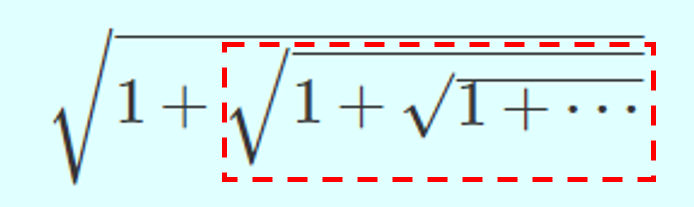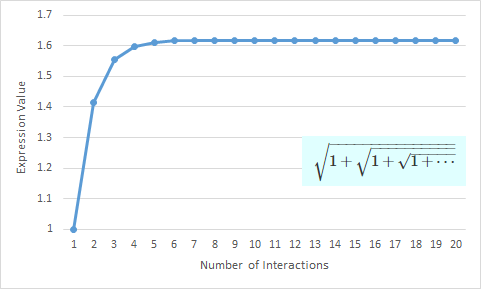#### Evaluate Recursive Expressions

Some recent daily problems relate to evaluating expressions with recursive patterns.

 (3245) Compute $$\frac{1}{\frac{1}{\frac{1}{\cdots}+1+\frac{1}{\cdots}}+1+\frac{1}{\frac{1}{\cdots}+1+\frac{1}{\cdots}}}$$

 (3882) Find the value of $$\sqrt{1+\sqrt{1+\sqrt{1+\cdots}}}$$

 (3254) Without using a calculator, explain that $$\sqrt{20+\sqrt{20+\sqrt{20}}}-\sqrt{20-\sqrt{20-\sqrt{20}}}\approx 1$$

There are several different techniques to solve such problems. One is to establish and then solve an equation. Let's use #3882 as an example. Because the pattern repeats infinitely, the value of the highlighted inner part will be the same as that of the whole expression!This insights lays the foundation of the of the equation based solution.

 (3246) Use at least two ways to prove $$\sqrt{x\sqrt{x\sqrt{x\sqrt{\cdots}}}}=x$$

GetSolution/3254

One chapter of the book is dedicated to tackling this type of problems. Here are a few more examples from that book.

 (3246) Use at least two ways to prove $$\sqrt{x\sqrt{x\sqrt{x\sqrt{\cdots}}}}=x$$

 (3249) Compute $$\sqrt{\frac{2}{2^2}+\sqrt{\frac{2}{2^4}+\sqrt{\frac{2}{2^8}+\cdots}}}$$

Sounds simple and intuitive? However, it is worth noting that this method is built upon an important assumption which is always implicitly held at middle/high school level: the given expression converges to a single finite value when the pattern repeats infinitely.

Let's still take #3882 as an example. If we calculate a few iterations and plot the values in a graph, it is intuitive to see that the value of this expression indeed approaches a fixed finite value.

$$\begin{array}{rcl} \sqrt{1}&=&1\\ \sqrt{1+\sqrt{1}}&=&1.4142... \\ \sqrt{1+\sqrt{1+\sqrt{1}}}&=&1.5537...\\ &\cdots& \end{array}$$To strictly prove a given infinite series converges to a fixed value is a subject of study in calculus. Therefore, it is beyond the scope of middle/high school competitions. However, understanding this implicit assumption will help us explain the following puzzle:

 (3928) John uses the equation method to evaluate the following expression:$$S=1-1+1-1+1-\cdots$$ and get $$S=1-S \implies \boxed{S=\frac{1}{2}}$$However, $S$ clearly cannot be a fraction. Can you point out what is wrong here?